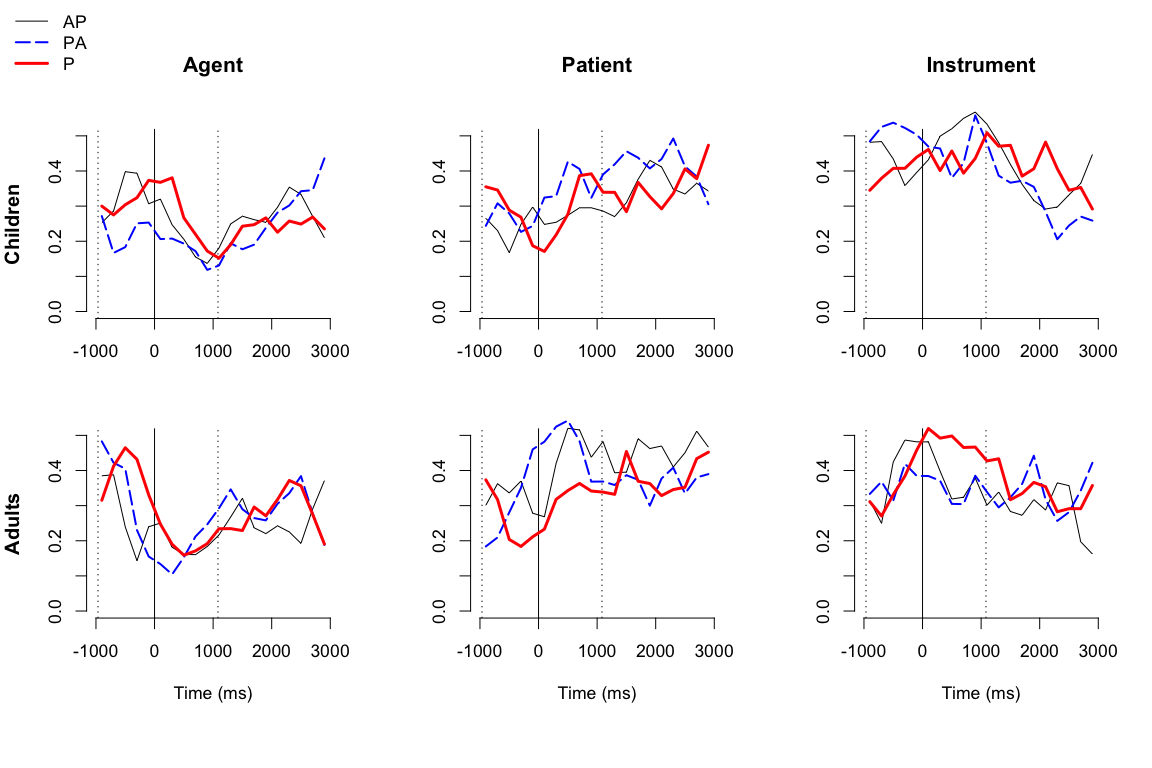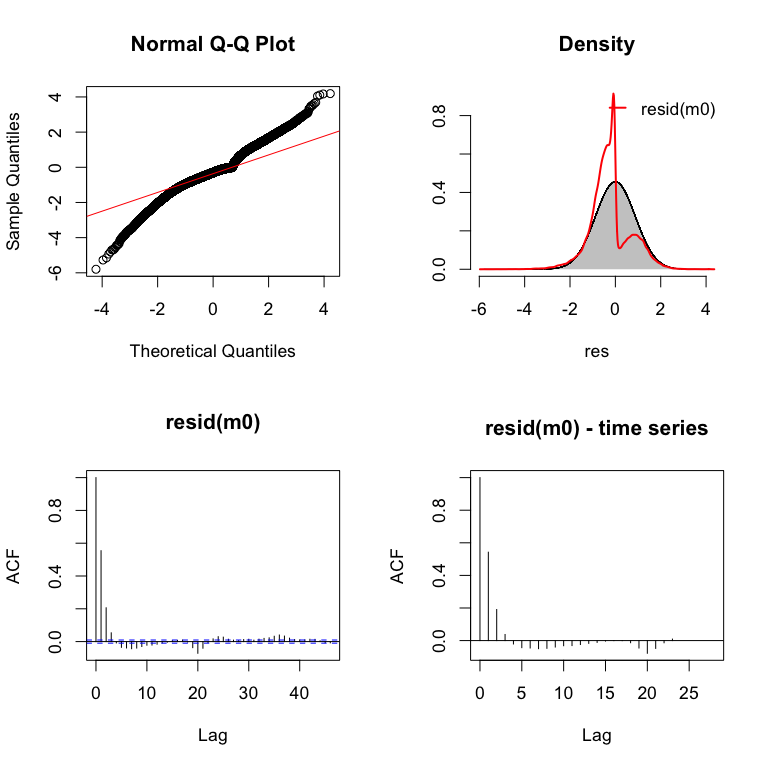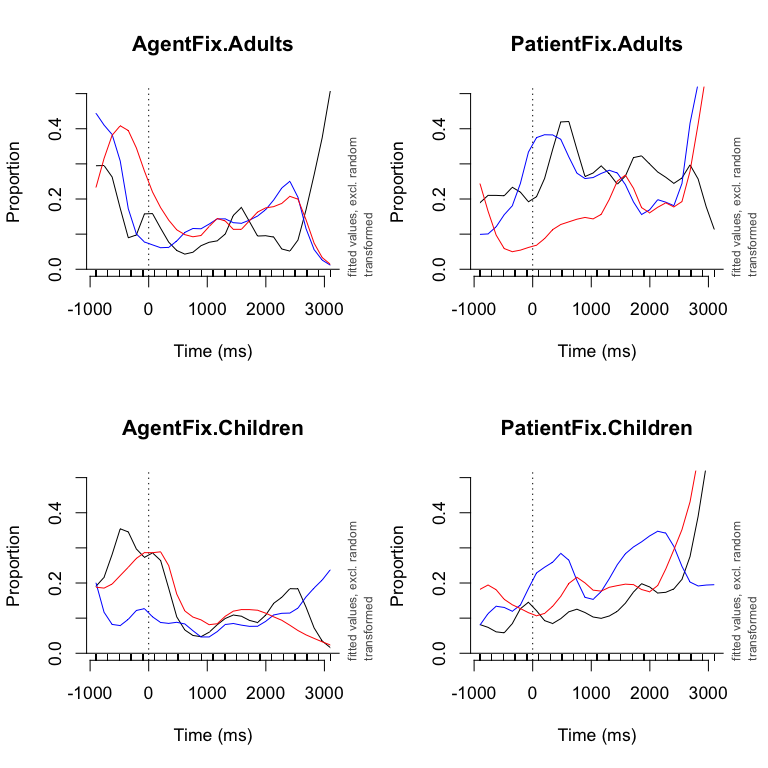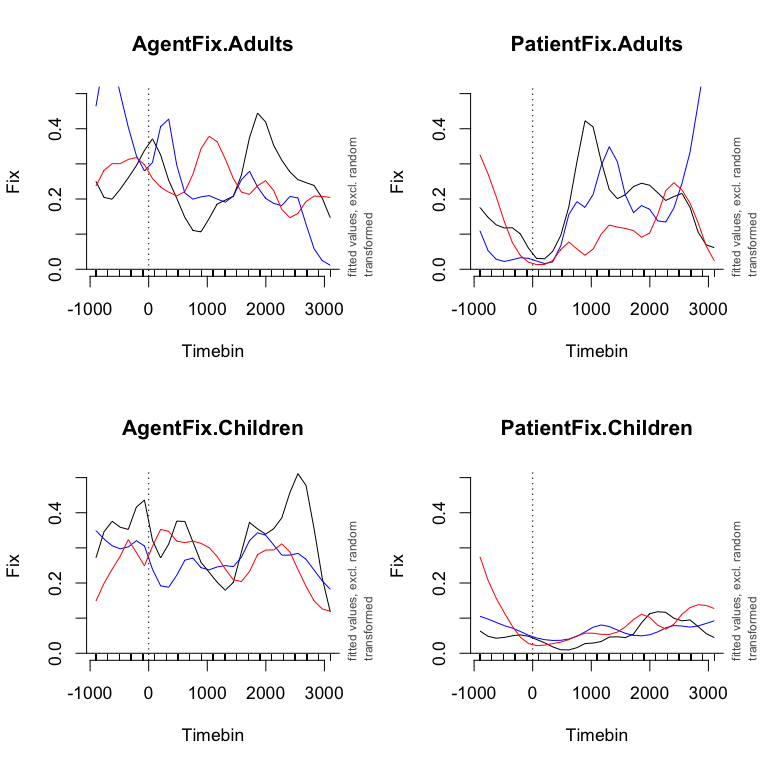#### contact: vanrij.jacolien `"at"` gmail `"dot"` com

Here the data, graphs and analyses scripts (R code) are provided for the paper:
Using context to resolve object pronouns, Jacolien van Rij, Bart Hollebrandse, and Petra Hendriks

Submitted for publication in:
Empirical perspectives on anaphora resolution: Information structural evidence in the race for salience, edited by Anke Holler, Christine Goeb and Katja Suckow.

Note: The code for the eye-tracking GAMM analyses is outdated due to updates in the packages mgcv and itsadug. See Porretta, Kyröläinen, van Rij, & Järvikivi (2017) for a more recent description of a GAMM analysis of VWP gaze data.

\(\leftarrow\) Experiment

\(\leftarrow\) Response data analysis

Gaze data analysis

# Gaze data

## 1. Data

Participants had to indicate whether the sentence they heard was a correct description of the picture presented on the screen. The responses were yes, no, or missing (labeled as NA).

The response data is available here. Loading data and packages in R:

``````# Gaze data:

# Although the experiment did not have many trials, the data is still relatively large:
dim(dat)``````
``##  488764     33``

``R.version.string``
``##  "R version 3.2.2 (2015-08-14)"``
``````# For GAMMs:
library(mgcv)``````
``````## Loading required package: nlme
## This is mgcv 1.8-7. For overview type 'help("mgcv-package")'.``````
``````# For GAMM interpretation and visualization:
``## Loaded package itsadug 1.0.1 (see 'help("itsadug")' ).``
``````# for generating this R Markdown report the
# info messages are put on:
infoMessages('on')
# load package MASS for calculating inverse later:
library(MASS)
# load package plyr for calculating averages:
library(plyr)
# For printable plot colors:
library(sp)
# Set plot colors:
col1 <- bpy.colors(4)
col2 <- bpy.colors(4)
col3 <- bpy.colors(4)
col4 <- bpy.colors(4)``````

Note: The GAMM analysis was performed with `mgcv` version 1.8-3, but this report and the plots were generated with a newer version of `mgcv` installed.

### Preprocessing

We classified the gaze positions as looks to the `agent`, looks to the `patient`, looks to the `instrument`, and `other` looks. To simplify analysis, we ignored the looks to empty parts of the screen (`other`). As we were interested in looks to the referents, we compared the number of looks to the `agent` with the total number of looks (i.e., the sum of the looks to the `agent`, `patient` and `instrument`), and we also compared the number of looks to `patient` with the total number of looks.

The function `getCountData` was used to count the fixations on each AOI per time bin of 200 ms. These counts were subsequently transformed to cbinds of successes and failures for each measure (i.e., looks to agent and looks to patient). Most logistic regression methods accept such two-count value data as input.

• The data frame is smaller (less rows), because the data is aggregated over time bins. This reduces the time to run a statistical model in comparison with raw binomial data (0 and 1).

• Aggregating over timebins reduces the correlation between subsequent measures in comparison with raw binomial data. In a VWP experiment, time bins of 100-200 ms are reasonable, because it takes about 200 ms to plan a saccade.

Alternatively, one could use the counts to calculate the logit or proportion of looks to an AOI before analyzing the data. The data is treated as Gaussian data. The advantage is that normal regression models are much faster than the logistic regression models. However, there are two disadvantages in comparison with the ‘cbind’ method:

• Information about the sample size underlying the values is lost when calculating a proportion or logit or other measures. The size of the counts in the ‘cbind’ provides a measure of certainty.

• When transforming the binary data to normally distributed measures, one does not take into account that the structure of the variance is not similar to a normal distribution, because the underlying distribution is binomial.

Based on these arguments, we decided to analyze count data. In the script below the fixations on the areas of interest are counted and converted to counts of successes and failures.

``````# Bin data in 200 ms time bins, and count fixations
dat\$Timebin <- timeBins(dat\$Time, 200)

# Count all AOIs in each timebin:
table(dat\$AOI)

cdat <- ddply(dat,
c('RecordingName', 'Subject', 'AgeGroup', 'Item',
'TrialCounter','imageType','Match','ReferringExpression',
'Context', 'Timebin', 'StartSentence1', 'Referent1',
'Referent2', 'EndSentence1', 'StartSentence2',
'StartAnaphor', 'EndAnaphor', 'StartPP', 'EndSentence2'),
summarize,
AOI.actor   = length(AOI[AOI=='actor' & !is.na(AOI)]),
AOI.patient = length(AOI[AOI=='agent2' & !is.na(AOI)]),
AOI.instr   = length(AOI[AOI=='instrument' & !is.na(AOI)]),
AOI.other   = length(AOI[AOI=='other' & !is.na(AOI)]),
AOI.NA      = sum(is.na(AOI)),
AOI.n       = length(AOI))

## Make long data:

# Create cbind of actor vs (agent2+instr):
dat1 <- cdat
dat1\$Fix = cbind(success=dat1\$AOI.actor,
failure= dat1\$AOI.patient+dat1\$AOI.instr)
dat1\$measure <- 'actorFix'

# Create cbind of patient vs (actor+instr):
dat2 <- cdat
dat2\$Fix = cbind(success=dat2\$AOI.patient,
failure=dat2\$AOI.actor+dat2\$AOI.instr)
dat2\$measure <- 'agent2Fix'

# combine
dat1 <- rbind(dat1, dat2)

# Create cbind of instr vs (actor+patient):
dat2 <- cdat
dat2\$Fix = cbind(success=dat2\$AOI.instr,
failure=dat2\$AOI.actor+dat2\$AOI.patient)
dat2\$measure <- 'instrFix'

# combine
dat1 <- rbind(dat1, dat2)

# order data:
dat1 <- dat1[order(dat1\$Subject, dat1\$Trial, dat1\$measure, dat1\$Timebin),]
rm(list=c('dat2'))

# define interactions:
dat1 <- dat1[dat1\$ReferringExpression=="P",]

dat1\$im <- sprintf("%sOim",toupper(substr(dat1\$imageType,1,1)))
dat1\$Factor <- with(dat1, interaction(Context,AgeGroup,measure,im))
dat1\$fixSubj <- with(dat1, interaction(measure, Subject))
dat1\$fixItem <- with(dat1, interaction(measure, Item))
dat1\$fixRec <- with(dat1, interaction(measure, RecordingName))

dat1\$TrialCounter <- as.numeric(as.character(dat1\$TrialCounter))
dat1\$Timebin <- as.numeric(as.character(dat1\$Timebin))
dat1 <- droplevels(dat1)

# Important: always sort data
dat1 <- dat1[order(dat1\$Subject, dat1\$Trial, dat1\$measure, dat1\$Timebin),]
# save data
save(dat1, file='Data/analysisdat.rda')``````

### Visualizing the data

For visualizing the data, the counts are converted to proportions:

``````# Calculate prop. looks to target versus other looks
dat1\$fixProp <- with(dat1,
ifelse((Fix[,1]+Fix[,2])==0, NA, Fix[,1] / (Fix[,1]+Fix[,2])) )
# aggregate, collapsing over trials:
fix <- with(dat1,
aggregate(fixProp,
list(Subject=Subject, Timebin=Timebin, Factor=Factor),
function(x){mean(x, na.rm=T)}) )
# aggregate, subject means:
fix.m <- with(fix[fix\$Timebin < 3000,],
aggregate(x,
list(Timebin=Timebin, Factor=Factor),
function(x){mean(x, na.rm=T)}))``````

Figure 4 in the paper plots the proportion looks to the AOI (agent, patient and instrument) for the three contexts (AP, PA, P) for the two age groups (adults and children) with a congruent picture (other-oriented action). The R code for the first plot is given below.

``````par(mfrow=c(2,3), cex=1.1)

# Get information about sound files:
info <- dat1[,c('RecordingName', 'Context', 'Referent1', 'Referent2', 'StartSentence2', 'StartAnaphor', 'EndSentence2')]
info <- info[!duplicated(info),]
a <- median(info\$StartSentence2-info\$StartAnaphor)
b <- median(info\$EndSentence2-info\$StartAnaphor)

# Plot settings
colors <- c('black', 'blue','red')
ltys <- c(1,5,1)
lwds <- c(1,2,3)
im <- 'OOim'

# Create plot window
emptyPlot(c(-1000,3000), .5,
main='Agent', xlab='', ylab='Children' )
abline(v=c(0, a, b), lty=c(1,3,3))

# plot Children, Agent looks:
group = "Children"
aoi   = "actor"

# plot lines:
i <- 1
for(context in c('AP', "PA", "P")){
# conditions:
condition <- paste(context, group, paste(aoi, 'Fix', sep=''), im, sep='.')

# data:
tmp <- fix.m[fix.m\$Factor==condition,]
tmp <- tmp[order(tmp\$Timebin),]
# plot lines:
lines(tmp\$Timebin, tmp\$x,
col=colors[i], lwd=lwds[i], lty=ltys[i], xpd=T)
i <- i+1
}``````Figure 5 in the paper, which plots the incongruent trials, follows the same code as for Figure 4. Only the image is replaced: `im <- "SOim"`.## 2. Analysis

The looks to the Agent and Patient are combined in a single analysis. Note that looks to the Agent and Patient are not completely dependent on each other: if the looks to the agent increase, necessarily the looks to other AOIs decrease. To reduce the dependency between Agent and Patient looks, we also counted the looks to the instrument, and included these in the failures of the Agent and Patient looks. However, we did not include the intrument looks as measure in our analysis.

``````dat1 <- dat1[dat1\$measure != "instrFix", ]
dat1 <- droplevels(dat1)
levels(dat1\$Factor)``````
``````##   "AP.Adults.actorFix.OOim"    "P.Adults.actorFix.OOim"
##   "P.Children.actorFix.OOim"   "PA.Children.actorFix.OOim"
##  "P.Children.agent2Fix.OOim"  "PA.Children.agent2Fix.OOim"
##  "P.Children.actorFix.SOim"   "PA.Children.actorFix.SOim"
##  "P.Children.agent2Fix.SOim"  "PA.Children.agent2Fix.SOim"``````

In the SDT analyses we used three analysis methods to test for the effects of Context:

1. Using a model-comparison procedure;

2. Inspection of the summary with difference curves with contrast coding (cf. Masson and Kliegl 2013);

3. Using plots of the model predictions.

These measures resulted in similar conclusions with respect to the effect of Context. Logistic regression takes much longer to run than regression on Gaussian data. With more than 60.000 data points in the reduced data, each model will still take some hours to run. Therefore, we skip the first two method and use the full model and the model predictions to estimate which effects are significant.

The full model is presented below. The model is available here.

``````# As the models take a really long time to run,
# we only consider the full model:
m0 <- bam(Fix ~ Factor
# general effect of all conditions, which does not change over time
+ s(Timebin, by=Factor, k=20)
# change over time in trial for each condition
+ s(Timebin, fixSubj, bs='fs', m=1)
# random effect: change over time for each Subject and AOI combination
+ s(Timebin, fixItem, bs='fs', m=1)
# random effect: change over time for each Item and AOI combination
+ s(fixRec, bs='re'),
# random intercept for each individual event (unique time series)
data=dat1, family='binomial')
save(m0, file='m0.rda')``````

Summary of the model. Note that the lines in the summary only tell whether the lines are significantly different from 0.

``gamtabs(m0, type='HTML')``

Residuals of the model are not normally distrubuted (which is also not required for logistic data). There is still some autocorrelation left, but that is difficult to reduce: adding random effects will increase the time for running the model (which was already quite long), and we cannot include an AR1 model for logistic GAMMs as is possible for normal GAMMs.

``check_resid(m0, split_by=list(dat1\$Subject))``### Method c: visualizing the model predictions

The R code for plotting the proportions looks to the agent (left panels) and patient (right panels) for congruent trials (other-oriented picture):

``````main=NULL

par(mfrow=c(2,2), cex=1.1)

# loop over AgeGroup:
# loop over Area of Interest (Agen, Patient):
for(aoi in c('Agent','Patient')){
# loop over context:
for(con in c("AP", "PA", "P")){
# make new plot for "AP":
if(con=="AP"){
main=paste(paste(aoi, "Fix", sep=''),ag, sep=".")
}
plot_smooth(m0, view='Timebin',
cond=list(Factor=paste(con,ag,paste(aoi, "Fix", sep=''),"OOim", sep=".")),
col=ifelse(con=='AP','black',ifelse(con=='PA', 'blue', 'red')), se=0,
main=main, ylab='Proportion', xlab='Time (ms)', ylim=c(0,.5),
}
}
}``````The same code was used for plotting the incongruent trials.Figure 6 in the paper combines the plots that visualize the looks to the patient (all four right plots).

### Visualizing predicted differences between conditions

Because we used factors instead of difference curves, the summary only tells whether each level is significantly different from zero (flat line), but not whether these levels are different from each other. We used the model predictions to calculate the differences between levels using the functions `get_difference` and `find_difference`.

#### i. Differences between age groups

Below the differences between adults and children (with 95%CI) are plotted for the congruent trials (other-oriented picture). The R code is also given. The differences that are significant are marked in red. These areas also occur in Figure 6 in the paper as intervals, but note that in Figure 6 we only focus on 0-1000 ms time window as indicated by the dashed vertical lines in the plots below.

``````old <- par()\$mar
par(mfrow=c(2,3), cex=1.1)

# loop over Area of Interest (Agen, Patient):
for(aoi in c('Agent','Patient')){
# change margins
if(aoi=="Agent"){
par(mar=old+c(-2,0,2,0))
}else{
par(mar=old+c(2,0,-2,0))
}
# loop over context conditions:
for(con in c("AP", "PA", "P")){
# calculate difference between adults and children:
newd <- get_difference(m0,
comp=list(Factor=paste(con, c("Adults", "Children"), paste(aoi, "Fix", sep=''),"OOim",sep=".")),
cond=list(Timebin=seq(-1000,3000, length=100)),
rm.ranef=TRUE)
# label in data frame newd which rows are significant:
newd\$f <- find_difference(newd\$difference, newd\$CI, as.vector=TRUE)

# setup plot:
emptyPlot(c(-1000, 3000), c(-3,3), h0=0, v0=c(0,1000),
main=ifelse(aoi=='Agent', con, "") )
if(aoi=="Agent"){
}else{
mtext('Timebin (ms)', side=1, line=3)
}
if(con=='AP'){
mtext(aoi, side=2, line=3, font=2, cex=1.25)
}

# fill significant area:
fill_area(newd\$Timebin, ifelse(newd\$f==FALSE, 0, ifelse(newd\$difference > 0, newd\$difference-newd\$CI, newd\$difference+newd\$CI)), from=0,
col='red', alpha=1, density=30)
# plot line + CI:
with(newd, plot_error(Timebin, difference, CI))
}
}``````